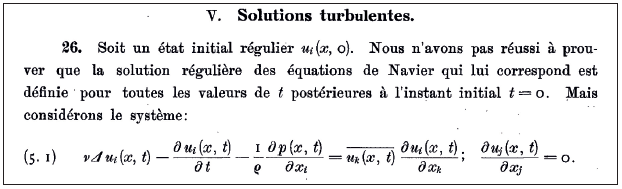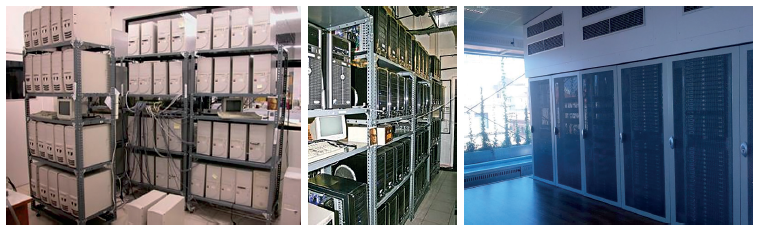# Numerical simulation and validation-verification of indoor fluid dynamics and heat transfer

ASSENSI OLIVA, C.D. PÉREZ SEGARRA, F. XAVIER TRIAS, JOAQUIM RIGOLA of the Heat and Mass Transfer Technological Center of the Universitat Politecnica de Catalunya (CTTC UPC)

This article was originally published in CLIMA NOTICIAS – Cluster IAQ. It analyses Computational Fluid Dynamics and Heat Transfer (CFD&HT) as an essential tool in the optimal design of HVAC systems to predict and determine indoor air quality (IAQ).

An essential aspect of the optimal design of HVAC systems is the prediction/determination of indoor air quality. This involves being able to predict the fields of speed, temperature, humidity and concentration/dissemination of species and particles. Prediction of these fields, these dependent variables, with sufficient precision would be equivalent to having a hypothetical laboratory that determines in detail the movement and quality of air from point to point and instant to instant.

### THE ORIGINS OF CFD

Based on this potential, various design options can be considered systematically: geometries, spatial and temporal contour conditions, etc. and the optimal designs can be selected for each situation. This is possible and immediate if we have the analytic solution to energy, mass and movement conservation equations, that is, the Navier-Stokes equations. This is a system of differential equations with partial, non-linear derivatives that are strongly coupled.

The analytical solution has only been achieved for highly simplified situations. The equations have been very useful to parameterise simple situations that are often present in HVAC systems and equipment. However, they do not enable simulation of all of the situations and conditions that are found in any installation, regardless of how simple it is.

From the 1960s to the 1970s, methods in Computational Fluid Dynamics & Heat Transfer (CFD&HT), which consist of subdividing the spaces to study into small finite volumes (FV) and requiring of each of them discrete (not infinitesimal) compliance with the aforementioned conservation equations, opens the door to being able to have in a discrete form (increment by increment in space and time if it is transitory) the fields of the dependent variables of interest to us.

The problem is that for the solution to be correct/accurate, very fine discretization tends to be necessary (millions, hundreds of millions of finite volumes, whatever the measurement, form and conditions of the contour: input and output flow of the domain).Navier-Stokes equations (in this case regularised) extracted from the famous article by Jean Leray “Sur le mouvement d’un liquide visqueux emplissant l’espace” Acta Mathematica. 63: 193–248 (1934). In this study, J. Leray demonstrated the existence and uniqueness of Navier-Stokes equations for two-dimensional cases. For three-dimensional cases, the problem remains open. In fact, it is one of the seven-millennium problems proposed by Clay Mathematics Institute in 2000.

### USE OF PARALLEL COMPUTERS

The first computers to try to do what is now known as high-performance computing (HPC), the first CRAYs (a lot slower than a current laptop), were promoted to resolve these equations numerically. Unfortunately, they were not used for HVAC or other issues that we consider beneficial for our wellbeing, but for military uses.

When PCs appeared, an intriguing, exciting situation emerged towards the end of the 1990s: for several years, some university environments constructed clusters of PCs with a calculation potential an order of magnitude higher than the latest generation CRAYs. It was then, at the start of 2000, that manufacturers that assembled processors (basically Intel and AMD) emerged, to configure a structure/technology such as current HPC infrastructures. The closest of these infrastructures in Catalonia are MareNostrum 4 and the small cluster at CTTC-UPC.

### MODELLING OF TURBULENCE

What we have been talking about is Direct Numerical Simulation (DNS), that is, solving Navier-Stokes equations numerically in all of their complexity (without any type of turbulence model). If we can make the discretization meshes sufficiently dense, this methodology gives us potential that is practically equivalent to what we would have if we had the analytical solutions to the equations.

Likewise, with the current calculation capacities, we can only solve small spaces with contour conditions of inflow at low speeds. This is the case in all CFD&HT fields, including HVAC situations and aerodynamics, combustion and two-phase phenomena. Even with the continuous improvements in the calculation potential of our computers, this limitation will continue to exist in the coming decades.

Many CFD&HT simulations that are carried out have a certain degree of uncertainty because the meshes are not dense enough (basically a calculation problem) and/or because the numerical discretizations of the equations need to be simplified by sacrificing part of the physics that is present.

The current state of the art is focused on refining these simplifications to sacrifice the minimum amount of physics that is present so that with the minimum finite volume we have acceptable solutions. We are talking about different models of turbulence.

From greater to lesser levels of modelling, we can find in the first place a Reynolds Average Navier-Stokes type model (RANS), in which Navier-Stokes equations are resolved and averaged over time. These have a relatively low calculation cost (we can solve them using a laptop in minutes), but quite a high level of modelling and uncertainty, especially in typical HVAC applications. At the other extreme, we find the DNS simulations mentioned above, in which the calculation time could easily be days, weeks or months, using hundreds or thousands of CPUs in parallel  .

Clearly, these types of simulations are not feasible for HVAC applications, due to the calculation cost (just the cost of electricity in one simulation could be thousands of euros) and the execution time.

Now, this type of simulation is key to provide reference solutions to calibrate models of turbulence and/or to better understand the phenomenology of flow and which limitations the models could have.

Halfway between DNS and RANS we have what is known as large eddy simulation (LES). This is basically the same solution methodology as in DNS cases, but with a much less dense mesh. This is where the name LES comes from the idea that only the largest scales are solved. We could be talking about reductions in the number of FVs of three orders of magnitude and a reduction in the number of time steps of one order of magnitude.

Clearly, this reduction in spatial and temporal resolution of the problem implies that these smaller scales have to be modelled suitably. Here we are talking about various models with smaller scales of turbulence, in which the CTTC has vast experience in simulation and in the development of this type of models.

Even if a certain degree of modelling is demanded, these LES models function very well for a wide range of applications including HVAC. Even so, although they are increasingly penetrating the market in certain industrial applications, in many cases these types of simulations continue to be too expensive or slow to use in a routine way in the design phases of HVAC systems.

The main problem (in fact, the one that limits more extensive use) is the inherent need to adequately solve (almost as if it were a DNS) the regions close to the walls where boundary layers exist that may or may not separate, depending on the working conditions of the system (examples of this are what is known as the Coandă effect that we can observe in many ventilation systems and a very Catalan tradition: the ‘dancing egg’). The layers of the fluid (air or water) that are closest to the walls play a crucial role in the dynamics of these boundary layers.

The problem is that these fluid regions are extremely fine and the correct numerical resolution involves using FV (and time steps) that are extremely small, which has a high calculation cost. To mitigate the computational costs associated with resolving these flow regions closer to the walls, hybrid RANS-LES models have emerged (basically, the area close to the walls is solved with RANS while the other part of the domain is solved with LES) and wall-modelled LES (WMLES), in which instead of solving these areas close to the wall, they are modelled using boundary layer models.Supercomputer Fugaku is situated in the RIKEN Center for Computational Science of Kobe (Japan). Currently, it is the most powerful supercomputer in the world according to the Top-500 ranking.Different configurations of the cluster of PCs JFF in the installations of CTTC (period: 1999-2010) and the current configuration (period 2011-2021).

### THE MAIN CHALLENGES OF THE FUTURE

Currently, we have a wide range of CFD methodologies, from RANS models to DNS simulations. All of these are extremely useful as long as they are used in the right context and with the knowledge that is required of the user. At CTTC, we have worked and continue to work on all levels of simulation. We also work on the development and improvement of the numerical techniques that are behind these simulations.

It is not the same to solve a case with a conventional PC as it is to do so on a modern supercomputer using tens of thousands of processors. In addition, we should look to the future, which is full of challenges: the efficient use of GPUs to carry out numerical calculations, ARM-type processors (like the mobile phones that we all carry) are gaining ground rapidly (the new Japanese supercomputer Fugaku that uses this technology is currently the most powerful in the world).

These are the three major current challenges for CFD&HT: improving modelling, developing increasingly efficient hardware, and developing/adapting codes and numerical algorithms for this new hardware (mass calculation in parallel, use of GPUs, heterogeneous computing, etc.).

It is important to be prepared for all the future could hold and to know how to respond to the technological needs of HVAC applications by combining in a smart way all levels of simulation: including DNS, LES, etc.; 0-dimensional/1-dimensional models that due to their simplicity enable problems with multiple components to be addressed; and the use of machine learning techniques fed with data that are being generated in CFD&HT simulations.